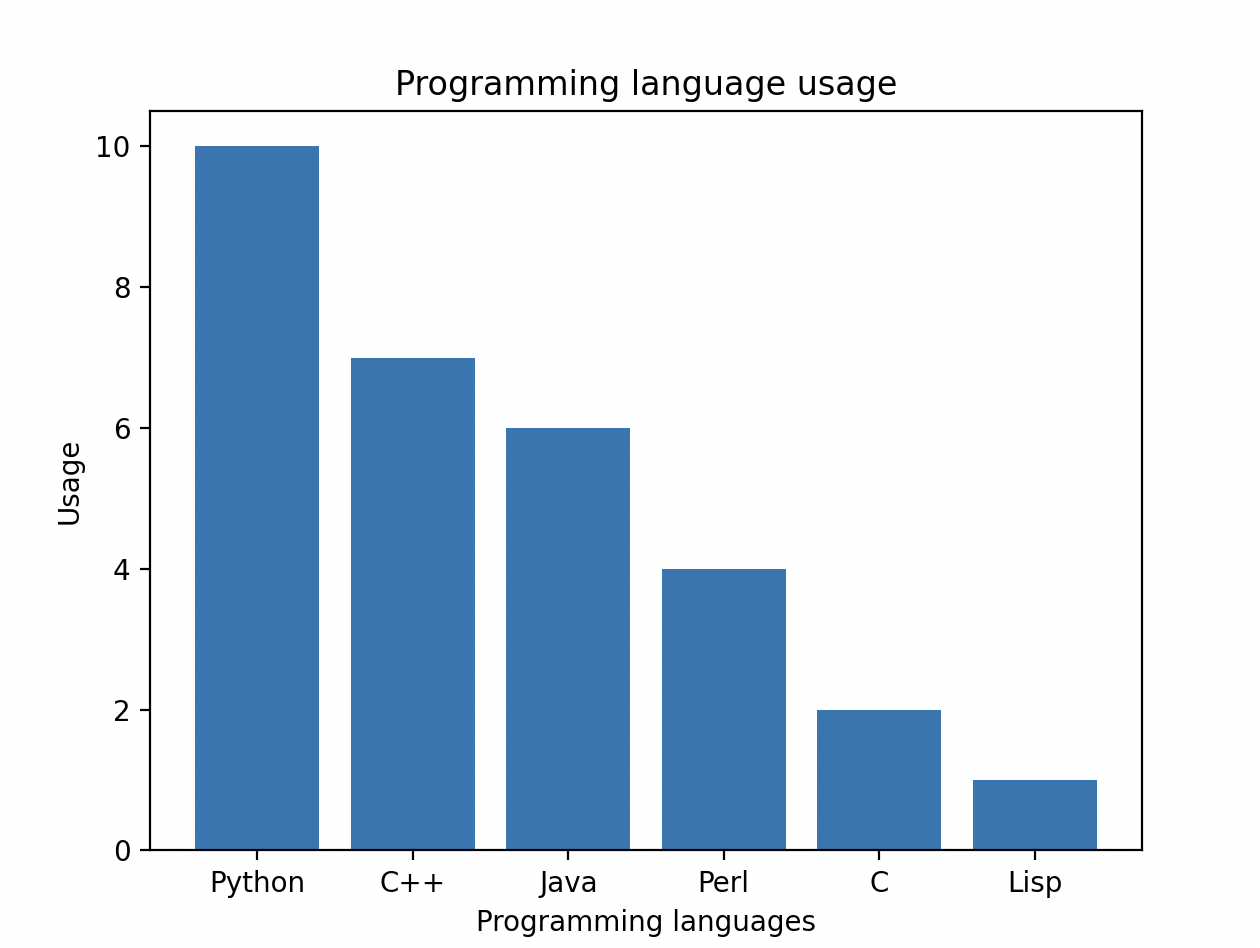# [Solved] Plot a bar chart to depict the popularity of various programming languages

1
Topic starter

Plot a bar chart to depict the popularity of various programming languages

Topic Tags
1
Topic starter

Code:

```import matplotlib.pyplot as plt
import numpy as np

prog_lanugages = ('Python', 'C++', 'Java', 'Perl', 'C', 'Lisp')

x = np.arange(len(prog_lanugages))

performance = [10,7,6,4,2,1]

plt.bar(x, performance)

plt.xticks(x, prog_lanugages)
plt.ylabel('Usage')
plt.xlabel("Programming languages")
plt.title('Programming language usage')
plt.show()```

Output: